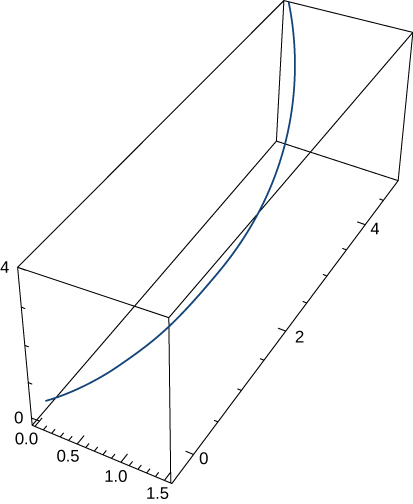Skip to main content
$$\newcommand{\id}{\mathrm{id}}$$ $$\newcommand{\Span}{\mathrm{span}}$$ $$\newcommand{\kernel}{\mathrm{null}\,}$$ $$\newcommand{\range}{\mathrm{range}\,}$$ $$\newcommand{\RealPart}{\mathrm{Re}}$$ $$\newcommand{\ImaginaryPart}{\mathrm{Im}}$$ $$\newcommand{\Argument}{\mathrm{Arg}}$$ $$\newcommand{\norm}{\| #1 \|}$$ $$\newcommand{\inner}{\langle #1, #2 \rangle}$$ $$\newcommand{\Span}{\mathrm{span}}$$

# 13.5E: Exercises for Section 13.5

$$\newcommand{\vecs}{\overset { \rightharpoonup} {\mathbf{#1}} }$$ $$\newcommand{\vecd}{\overset{-\!-\!\rightharpoonup}{\vphantom{a}\smash {#1}}}$$$$\newcommand{\id}{\mathrm{id}}$$ $$\newcommand{\Span}{\mathrm{span}}$$ $$\newcommand{\kernel}{\mathrm{null}\,}$$ $$\newcommand{\range}{\mathrm{range}\,}$$ $$\newcommand{\RealPart}{\mathrm{Re}}$$ $$\newcommand{\ImaginaryPart}{\mathrm{Im}}$$ $$\newcommand{\Argument}{\mathrm{Arg}}$$ $$\newcommand{\norm}{\| #1 \|}$$ $$\newcommand{\inner}{\langle #1, #2 \rangle}$$ $$\newcommand{\Span}{\mathrm{span}}$$ $$\newcommand{\id}{\mathrm{id}}$$ $$\newcommand{\Span}{\mathrm{span}}$$ $$\newcommand{\kernel}{\mathrm{null}\,}$$ $$\newcommand{\range}{\mathrm{range}\,}$$ $$\newcommand{\RealPart}{\mathrm{Re}}$$ $$\newcommand{\ImaginaryPart}{\mathrm{Im}}$$ $$\newcommand{\Argument}{\mathrm{Arg}}$$ $$\newcommand{\norm}{\| #1 \|}$$ $$\newcommand{\inner}{\langle #1, #2 \rangle}$$ $$\newcommand{\Span}{\mathrm{span}}$$

## Finding Components of Acceleration & Kepler's Laws

1) Find the tangential and normal components of acceleration for $$\vecs r(t)=t^2\,\hat{\mathbf{i}}+2t \,\hat{\mathbf{j}}$$ when $$t=1$$.

Answer:
$$a_\vecs{T}=\sqrt{2}, \quad a_\vecs{N}=\sqrt{2}$$

In questions 2 - 8, find the tangential and normal components of acceleration.

2) $$\vecs r(t)=⟨\cos(2t),\,\sin(2t),1⟩$$

3) $$\vecs r(t)=⟨e^t \cos t,\,e^t\sin t,\,e^t⟩$$. The graph is shown here:Answer:
$$a_\vecs{T}=\sqrt{3}e^t, \quad a_\vecs{N}=\sqrt{2}e^t$$

4) $$\vecs r(t)=⟨\frac{2}{3}(1+t)^{3/2}, \,\frac{2}{3}(1-t)^{3/2},\,\sqrt{2}t⟩$$

5) $$\vecs r(t)=\left\langle 2t,\,t^2,\,\dfrac{t^3}{3}\right\rangle$$

Answer:
$$a_\vecs{T}=2t, \quad a_\vecs{N}=2$$

6) $$\vecs r(t)=t^2\,\hat{\mathbf{i}}+t^2\,\hat{\mathbf{j}}+t^3\,\hat{\mathbf{k}}$$

7) $$\vecs r(t)=⟨6t,\,3t^2,\,2t^3⟩$$

Answer:
$$a_\vecs{T}=\dfrac{6t +12t^3}{\sqrt{1+t^2+t^4}}, \quad a_\vecs{N}=6\sqrt{\dfrac{1+4t^2+t^4}{1+t^2+t^4}}$$

8) $$\vecs r(t)=3\cos(2πt)\,\hat{\mathbf{i}}+3\sin(2πt)\,\hat{\mathbf{j}}$$

Answer:
$$a_\vecs{T}=0, \quad a_\vecs{N}=12\pi^2$$

9) Find the tangential and normal components of acceleration for $$\vecs r(t)=a\cos(ωt)\,\hat{\mathbf{i}}+b\sin(ωt)\,\hat{\mathbf{j}}$$ at $$t=0$$.

Answer:
$$a_\vecs{T}=0, \quad a_\vecs{N}=aω^2$$

10) Suppose that the position function for an object in three dimensions is given by the equation $$\vecs r(t)=t\cos(t)\,\hat{\mathbf{i}}+t\sin(t)\,\hat{\mathbf{j}}+3t\,\hat{\mathbf{k}}$$.

a. Show that the particle moves on a circular cone.

b. Find the angle between the velocity and acceleration vectors when $$t=1.5$$.

c. Find the tangential and normal components of acceleration when $$t=1.5$$.

Answer:
c. $$a_\vecs{T}=0.43\,\text{m/sec}^2, \quad a_\vecs{N}=2.46\,\text{m/sec}^2$$

11) The force on a particle is given by $$\vecs f(t)=(\cos t)\,\hat{\mathbf{i}}+(\sin t)\,\hat{\mathbf{j}}$$. The particle is located at point $$(c,0)$$ at $$t=0$$. The initial velocity of the particle is given by $$\vecs v(0)=v_0\,\hat{\mathbf{j}}$$. Find the path of the particle of mass $$m$$. (Recall, $$\vecs F=m\vecs a$$.)

Answer:
$$\vecs r(t)=\left(\dfrac{-\cos t}{m}+c+\frac{1}{m}\right)\,\hat{\mathbf{i}}+\left(\dfrac{−\sin t}{m}+\left(v_0+\frac{1}{m}\right)t\right)\,\hat{\mathbf{j}}$$

12) An automobile that weighs $$2700$$ lb makes a turn on a flat road while traveling at $$56$$ ft/sec. If the radius of the turn is $$70$$ ft, what is the required frictional force to keep the car from skidding?

13) Using Kepler’s laws, it can be shown that $$v_0=\sqrt{\dfrac{2GM}{r_0}}$$ is the minimum speed needed when $$\theta=0$$ so that an object will escape from the pull of a central force resulting from mass $$M$$. Use this result to find the minimum speed when $$\theta=0$$ for a space capsule to escape from the gravitational pull of Earth if the probe is at an altitude of $$300$$ km above Earth’s surface.

Answer:
$$10.94$$ km/sec

14) Find the time in years it takes the dwarf planet Pluto to make one orbit about the Sun given that $$a=39.5$$ A.U.

## Contributors

Gilbert Strang (MIT) and Edwin “Jed” Herman (Harvey Mudd) with many contributing authors. This content by OpenStax is licensed with a CC-BY-SA-NC 4.0 license. Download for free at http://cnx.org.

• Was this article helpful?